# Tangents and Normals

### From DT Online

##### Description

Tangents are lines just touching a given curve and its Normal is a line perpendicular to it at the point of contact (or point of Tangency).

Taking the common case of a Circle, the Normal to a Tangent from a point P on the circumference is a line joining the point to the circle centre - and the Tangent is at right angles to the Normal. This forms the basis for methods of constructing Tangents such that the Point of Tangency is given accurately.

##### To construct a Tangent to a Circle from a point outside its circumference:
• Join the point to the circle centre.
• Draw a semi-circle on this line as the diameter.
• The Point of Contact (or Tangency) for the Tangent is where the semi-cicle intersects the circle and its Normal joins this point to the circle centre (angle in semi-circle).

##### Construction of Tangents to an Ellipse

To construct a Tangent to an Ellipse from any point on the curve:

• Join the point to each of the two Foci as shown and bisect the angle found between the two lines.
• The bisector is the Normal and a Tangent may be drawn at right angles to it.

To construct a Tangent to an Ellipse from any point outside the curve:

• Strike an arc with the point as centre and radius equal to its distance from the Focus as shown.
• Strike a second arc with the second Focus as centre and radius set to equal the Major Axis.
• Join the points of intersection of the two arcs to the second Focus as shown.
• Where the two lines intersect the curve give the two Points of Tangency.
• Join the point to these Points of Tangency to produce the two possible Tangents and their Normals may be drawn at right angles to them.

##### Construction of Tangents to a Parabola

Three methods to construct a Tangent to a point on the Parabola

Method 1.

• Join the point to the Focus and draw from it a perpendicular to the Directrix.
• The bisector of the two lines created is the Tangent and its Normal may be constructed at right angles to it.

Method 2.

• Draw a line from the point perpendicular to the Axis (i.e. an Ordinate)
• With the Vertex as centre and radius the distance from the Vertex to where the Ordinate intersects the Axis, draw a semi-circle.
• Draw the Tangent to the point from where the semi-circle cuts the Axis as shown and its Normal may be constructed at right angles to it.

Method 3.

• Join the point to the Focus construct a line at right angles to it from the Focus.
• Draw the Tangent to the point from the line's intersection the Directrix and its Normal may be constructed at right angles to it.

##### To construct a Tangent from a point outside the Parabola
• Join the point (P) to the Focus(F) and using this as diameter, decribe a circle.
• Draw Tangent at the Vertex (V) perpendicular to the Axis to intersect the circle circumference in X and Y.
• Join PX and PY and produce both to touch the Parabola generating the two Tangents as shown.
• To draw a Normal, produce a Tangent (e.g. YP) to meet the Directrix in D and join D to the Focus(F).
• A line drawn perpendicular to DF will intersect the Parabola at a Point of Tangency(T).
• Construct a perpendicular to the Tangent from its Point of Tangency(T) to create its Normal.

##### Other terms common to all conics

Tangents and Normals are two terms used to describe properties of all conics. Other common properties are as follows:

• A Chord is a straight line joining two points on the curve.
• A Focal Chord is a Chord which passses through the Focus
• The mid-points of parallel Chords lie in a straight line called a Diameter
• A perpendicular from a point on the Axis is called an Ordinate and if it goes straight across to the other side, it is a Double Ordinate.
• The Double Ordinate through the Focus is the Latus Rectum (translates from Latin as ‘The Right Side’).
• A Normal to any conic is at right angles to a Tangent at the Point of Contact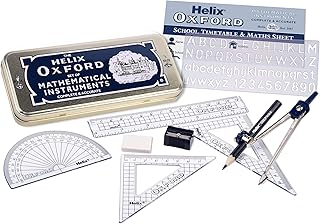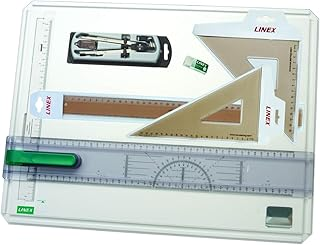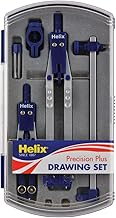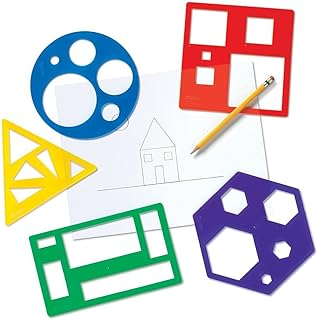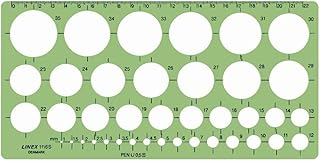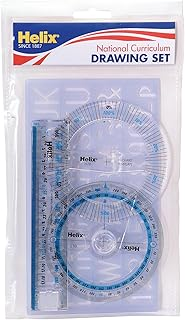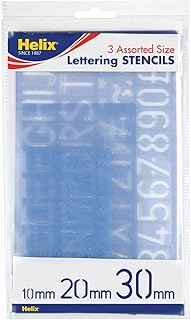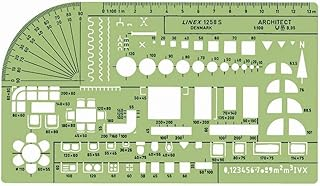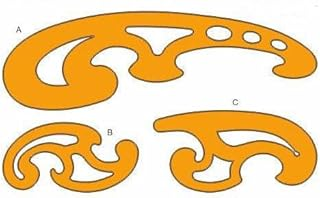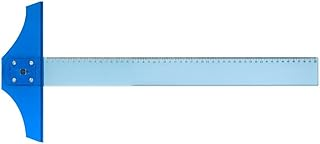Helix Oxford Maths SetLinex Student Saver BundleHelix Precision Plus Drawing SetPrimary Shape Template SetLinex Circle TemplateHelix National Curriculum Drawing SetHelix 3 Piece Stencil SetLinex Universal Architects TemplateFrench Curve Transparent TemplateT-Square